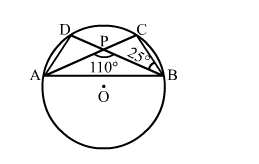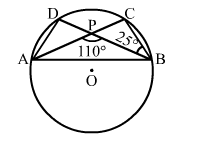# In the given figure, O is the centre of the circle. if ∠PBC = 25° and ∠APB = 110°,

Question:

In the given figure, O is the centre of the circle. if PBC = 25° and ∠APB = 110°, find the value of ∠ADB.Solution:

From the given diagram, we have:ACB = PCB
BPC = (180° - 110°) = 70°   (Linear pair)

Considering ΔPCB, we have:
PCB + BPC + PBC = 180°   (Angle sum property)
⇒ PCB + 70° + 25° = 180°
⇒ PCB = (180° – 95°) = 85°
⇒ ACB = PCB = 85°

We know that the angles in the same segment of a circle are equal.
∴ ADB = ACB = 85°